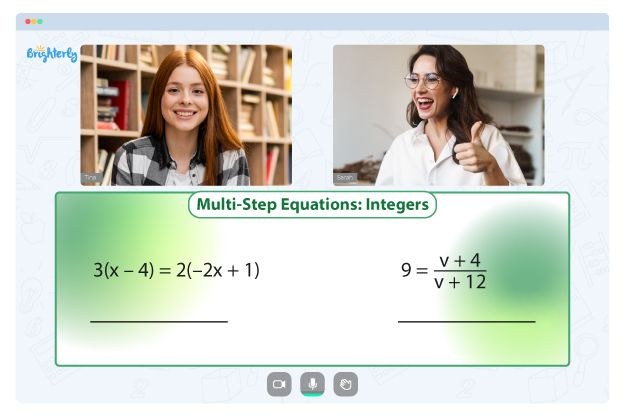# Rational Exponents Worksheets

Rational exponents worksheets help kids learn how to work with rational exponents. Teachers often have to break down the concepts to get students interested enough and assist them at becoming good at the topic. Worksheets on rational exponents can allow students to practice rational exponents at home to do better in tests and exams.

## Benefits of rational exponents worksheets

Here are some of the benefits of a rational exponents worksheet with answers:

### It Helps kids understand the concept of rational exponents

With a rational exponent worksheet, kids can repeatedly practice the topic at home until they understand it well enough. In addition, children can use a rational exponent worksheet with answers to know when they are wrong and right.

Math for Kids

Is Your Child Struggling With Math?
1:1 Online Math Tutoring### It helps kids remember the formulas to use

Using rational exponents worksheet answers helps kids repeat the formulas often and replicate them easily in exams and tests. If kids use the rational exponents worksheet answer key, they can learn when a formula they applied is wrong and what the right formula could be.### Rational Exponent Worksheet PDF

Rational Exponent Worksheet PDF### Rational Exponents Worksheet Answers PDF### Rational Exponents Worksheet with Answers PDF### Worksheets on Rational Exponents PDF

Worksheets on Rational Exponents

Struggling with Equations?• Start studying with an online tutor.

Is your child finding it challenging to grasp equations concepts? An online tutor could be the answer.